# Graphs

### Overview

A graph is a data structure that consists of a number of nodes (or vertices) and a number of edges. An edge is a connection between two nodes. Nodes are used to represent objects and the edges represent some kind of relationship between them. Below is a diagram of a graph with 6 nodes and 6 edges:Graphs are a very useful data structure as they can be used to represent many different kinds of relationships between data. There are many graph algorithms that operate on graphs, of which we will only cover a small number.

### Graph Applications

Graphs are used to represent many relationships between data. A few examples:

• Computer networks.
• Transportation.
• Compilers.
• Matching problems.
• 3D graphics.
• Relationships between information.

### Types of Graphs

A directed graph is one in which the edges have a specific direction. It is possible to have two edges connecting two nodes: one in one direction and another in the other. Below is a diagram of a directed graph:A weighted graph is one in which the edges have some number, called a weight, associated with them. One example where weighted graphs are used is to represent paths between different locations, where the distances between are given as weights. Below is a diagram of a weighted graph:A connected graph is one in which there is a path from any one node to every other. The graph above is not connected because there is no way to get from node 2 to node 3. Below is a diagram of a connected graph:A complete graph is one in which there is an edge between every pair of nodes in the graph. Below is an undirected complete graph with five nodes.For a directed graph to be considered complete, it would need two edges for every pair of nodes, one in each direction.

An acyclic graph is one in which there are no cycles. A cycle is a set of edges that can take you from a given node back to that node. The following graph has a cycle which is shown in red:Because the following graph has no cycles, it is called acyclic:A tree is a special type of directed graph which is acyclic and has the additional rules that one node (the root) has no edges entering it, and every other node has exactly one edge entering it: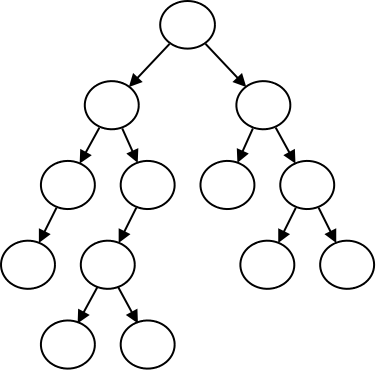One way to store a graph in a computer program is to use an adjacency matrix which is a two dimensional array. The matrix is $N \times N$ where $N$ is the number of nodes.

At each location in the matrix is stored the weight of the edge that connects those two nodes if there is one: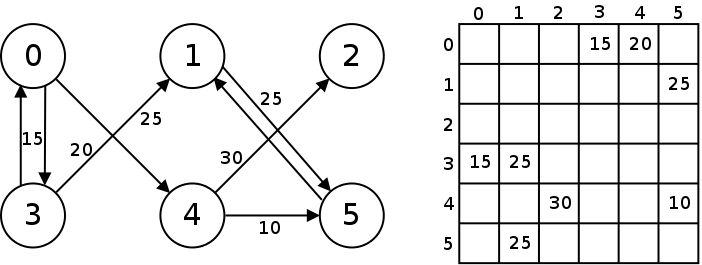For the locations in the adjacency matrix where there is no edge, we can store a sentinel value such as 0 or -1. In most applications weights that are zero or less would not be used (such as for distances).

For unweighted graphs, the values stored in the matrix can just be true or false.

This example shows a Graph class implemented using an adjacency matrix.

### Incidence List

An alternative way to implement a graph is with an incidence list. In an incidence list, we store a linked list for each node that contains the edges for that node. Each element in the linked list represents one edge in the graph, and stores the target node and the weight (if any).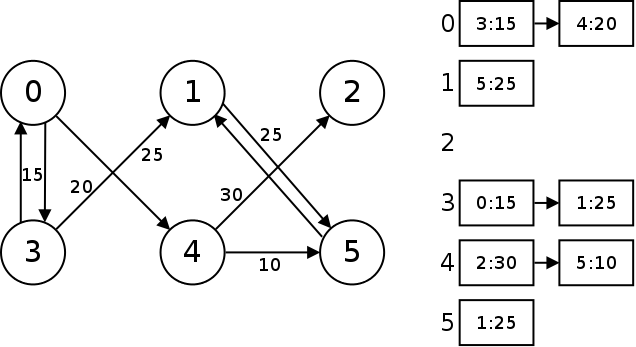To add an edge to the graph, we would go to the linked list associated with the node the edge is coming from, and insert a new edge in that list. To find whether or not an edge connects two nodes, and what the weight is, we have to search the list of edges.

This example shows a Graph class implemented using an incidence list.

Adjacency matrices and incidence lists provide different benefits. What are the trade-offs between the two approaches?

### Storing Data in the Graph

In the examples above, we have the nodes of a graph represented with integers from 0 to $N$ - 1. We then use either an adjacency matrix or incidence list to store the edges to other nodes. The examples above don't actually store any data in the graph nodes though.

To do that for an adjacency matrix, we would store a 1-dimensional array where each element stores the data associated with each node. We would still have the 2-dimensional array with the edges.

For an incidence list, we would have each node store the data for each node and the list of edges coming out of it together. We would then create a list or array of nodes to represent the graph. This is illustrated in this example.

### Finding Shortest Paths

One common problem is that of finding the shortest path between two nodes in a graph. This has applications in transportation and navigation such as GPS systems or Google Maps. It is also a problem faced by routers in a computer network when they decide how to route data across a network.

Below is an undirected, weighted graph representing several cities in Virginia along with approximate distances between them. Given a graph like this, we would like to find the shortest path between any two cities.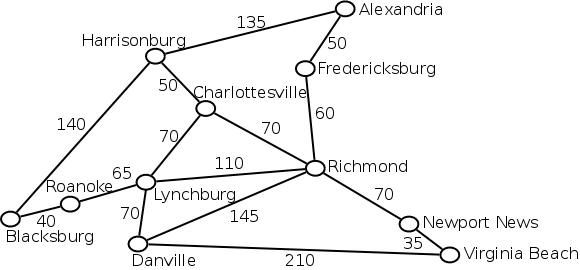The most common algorithm to solve this problem is called "Dijkstra's algorithm". The algorithm works by finding the distance from the start node to every other node in the graph. The algorithm starts by assuming that all of the distances are infinity. It then searches out and replaces these distances with better ones as it finds them. It does this by looping through the nodes, starting with the start node, and setting the neighbors distances to the distance to the current node plus the cost of the edge. When the distance to the end node is found, we are done. The algorithm is given below:

1. Set the tentative distance from the start node to all others to infinity.
2. Set the tentative distance from the start node to itself to 0.
3. Mark all the nodes as unvisited.
4. Set the current node to the start one.
5. While there is a current node:
1. For each neighbor of the current node:
1. If the neighbor's tentative distance is more than the sum of the distance to current + the cost of the edge from current, set the neighbors tentative distance to the distance to current + the cost of the edge from current to neighbor, and set the neighbor to unvisited.
2. Mark the current node as visited.
3. Set the current node to the unvisited node with the least tentative cost.
6. Return the tentative distance to the end node which is now minimal.

Dijkstra's algorithm is implemented in this example. Notice that we insert each edge in both directions because we assume each street is a two-way street.

What is the Big-O of this algorithm?





### Minimum Spanning Trees

A spanning tree is a tree that is made by selecting a subset of the edges of a graph such that all of the nodes of the graph are in the tree. The tree must also be a tree, so there can be no cycles in it. Below is a spanning tree for the cities graph: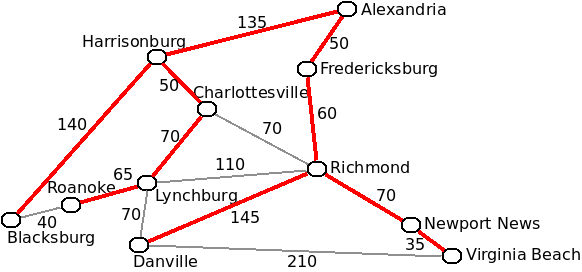Which node is considered to be the root is not important when discussing spanning trees. There can be multiple spanning trees for any one graph. The minimum spanning tree is the one for which the sum of the weights in the tree has the least value. Also note that only a connected graph can have a spanning tree.

There are several applications of minimal spanning trees. For example if an electric company wished to supply electricity for each of the above cities, running power lines along the edges of the minimal spanning tree will be use the least amount of cable.

There are several algorithms to solve this problem, one we will talk about is Prim's algorithm. Prim's algorithm starts with an empty minimal spanning tree. It then adds any one node to the tree. Then it repeatedly adds one node and one edge to the graph until it has a minimal spanning tree. Each time through it chooses the edge that connects a node in the tree with one not yet in the tree. If there are several, it chooses the one with the least weight.

This example implements Prim's algorithm. The minimal spanning tree that is actually found for this graph is depicted below: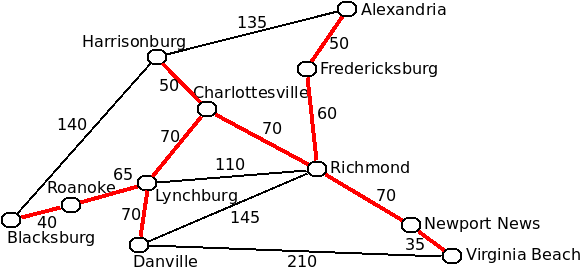What is the Big-O of this algorithm?





### The Travelling Salesman Problem

Another graph problem is the travelling salesman problem. In this problem, there is a salesman who would like to visit all of a set of cities in order to sell their products. To do this, they need to make a tour of the cities where they visit each city exactly once and end up back where they started.

It is not possible to complete a tour for every graph. For example in the graph of Virginia cities given above it is not possible to complete a full tour and end up back where we started. For the graph below, however it is possible: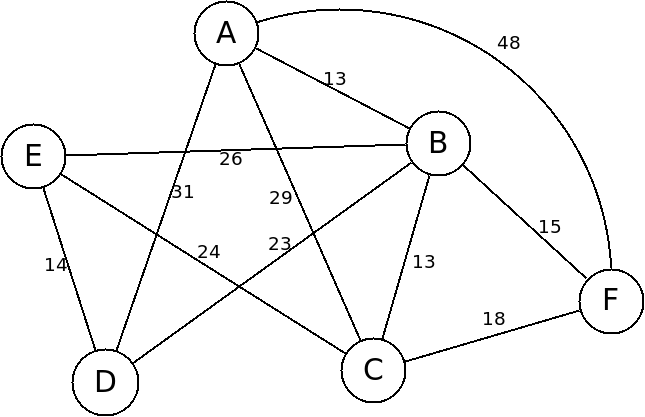One tour is C-F-A-B-D-E-C. Another is from A-B-F-C-E-D-A. However these tours will take different amounts of time to complete based off of the edges we must take on the tour. The first will take 148 and the second will take 115. Clearly the salesman will prefer to take the second tour as it will cost him less time, gas or airfare.

The travelling salesman problem is to find the tour with the least total cost.

The number of possible tours through the graph is the same as the number of permutations of the cities in the graph. A permutation is a specific order of the cities. There are $N!$ permutations of $N$ cities.

The direct approach to solving the problem is to generate all $N!$ permutations, calculate the cost of each one, and see which is the longest. This is called a brute force approach and is illustrated in this example. This program goes through the possible permutations recursively.

What is the Big-O of this algorithm?





The graph below shows how complexities increase for $N$: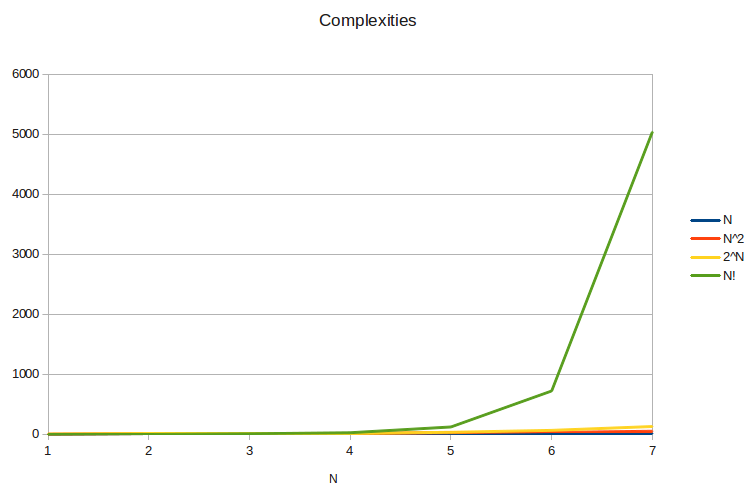As you can see, $N!$ completely dwarfs even $2^N$ as $N$ increases.

### Classes of Problems

In computer science, we classify problems in terms of how difficult they are to solve. Algorithms which have a Big-O complexity of the form $O(N^k)$ where $k$ is a constant are called polynomial problems. Even if an algorithm is $O(N^{1000})$ it is considered to be polynomial. Problems that have a polynomial algorithm are said to be in class $P$.

This means that the shortest path problem, the minimal spanning tree problem and most of the other problems we have looked at are in class $P$.

The travelling salesman problem has no known polynomial algorithm that can solve it. The best algorithm known is $O(N^2 \cdot 2^N)$. This problem belongs to a class of very hard programs called "$NP$-Complete" which have no polynomial time algorithms. Moreover, it's very unlikely that there even exists a polynomial algorithm to any $NP$-Complete problem. If you find one, you'll receive a $1,000,000 prize! Due to their open nature, graphs have several other$NP\$-Complete problems associated with them:

• Vertex Cover: Finding the minimum number of nodes that attach to each edge in the graph.
• Graph Coloring: Finding the minimum number of colors we can color the nodes such that no two of the same color are adjacent.
• Finding Cliques: Finding the larges complete subgraph (clique) in a graph.

These may sound esoteric, but many useful problems reduce to one of these difficult problems.

Scheduling problems can reduce to the graph coloring problem, as can the problem of allocating registers in a compiler.

The clique problem comes up in protein folding and gene modelling applications.

Being able to recognize these problems may keep you from spending time trying to solve an infeasible problem!